Next: CAUSALITY AND WAVE PROPAGATION Up: Spectral factorization Previous: WHITTLE'S EXP-LOG METHOD

# THE KOLMOGOROFF METHOD

If in a computer we have the coefficients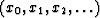of a polynomial X(Z), we say we are working in the time domain.'' If we evaluate the polynomial X(Z) at a number of positions on the unit circle, we have numbers, say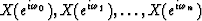,which we call a frequency-domain representation of the polynomial X(Z). We have seen that the fast Fourier transform is a very cheap way of going from the time domain to the frequency domain and back. This makes it very worthwhile to look at Whittle's factorization method in the frequency domain. Furthermore, we will understand spectral factorization from yet another point of view.

We may begin with a time function or Z transform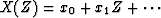.Let us denote by Xk the transform of the time function, that is, X(Z) evaluated at numerous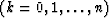places on the unit circle. Consider the identities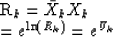Now we add and subtract a still arbitrary function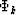to the exponential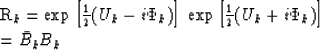Now the big question is whatshould be used to guarantee that Bk transforms to a minimum-phase, one-sided time function? By looking at Whittle's method, we note that the only significant properties of U+ (Z) are that it is finite and that the time function ut vanishes before t = 0. Thus we expect thatshould be chosen so that when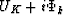is transformed into the time domain the resulting time function u+t should vanish for negative time. This may be done as depicted in Figure 4.

 3-4 Figure 4 Determination of the phase function.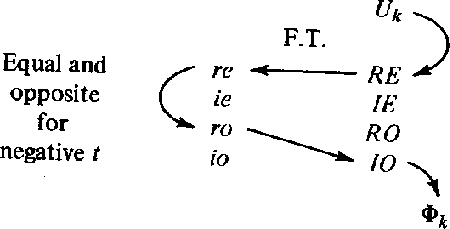To see how easy it really is to get the imaginary odd part IO, we fetch the integration filter from Sec. 2-8 (on bilinear transformation) and display it in Figure 5.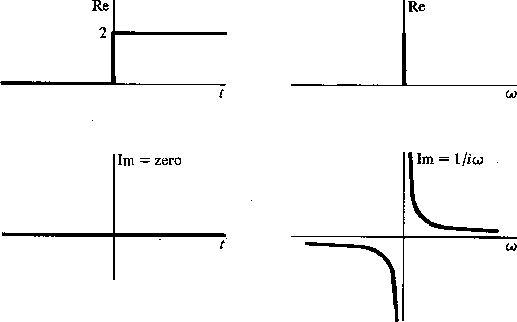3-5
Figure 5
The transform pair used in the Hilbert transform.To getwe take Uk into the time domain, getting ut. Then we multiply by the real step function of time in Figure 5, obtaining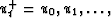.This implies that in the frequency domain Uk has been convolved with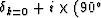phase shift filter). Thus,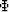has been generated.

Let us reconsider the operation of dropping all of the negative powers of Z in U(Z) as we did in the previous section to get U+ (Z). For simplicity, consider the case rt real; then ut is real.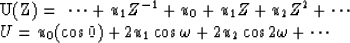Now let us make up a new functionby replacing cosine by sine in the foregoing expression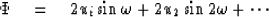We now see that combining U with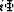we get U+.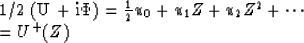Notice that the operation of changing cos t to sin t would be called 90phase shift filtering. Here we have changed cos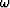to sinwith the result that U+ (Z) has only positive coefficients of Z.

The Kolmogoroff method of spectral factorization is very fast in a computer because fast Fourier transforms may be used. Its principle disadvantage is that summation around the unit circle is always slightly different than integration about the circle. When the spectrum is simple but poles are very close to the unit circle, then the Toeplitz method may prove more satisfactory. A simple program to do spectral factorization is given in Figure 6.

## EXERCISES:

1. Insert the additional arrows in Figure 4 which are required when dealing with complex time functions.
2. What is the meaning of minimum-phase waveform if the roles of time domain and frequency domain are interchanged?
3. Show how to do the inverse Hilbert transform, given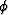find u. What is the interpretation of the fact that you cannot get u0?
4. Consider a model of a portion of the earth where x is the north coordinate, +z represents altitude above the earth, and magnetic bodies are distributed in the earth so as to create no magnetic field component in the east-west direction. One may show that the magnetic field h above the earth is represented by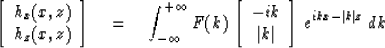Here F(k) is some spatial frequency spectrum.

(a)
By using Fourier transforms, how does one compute hx(x, 0) from hz (x, 0) and vice versa?
(b)
Given hz(x, 0), how does one compute hz(x, z)?

(c)
Notice that at z = 0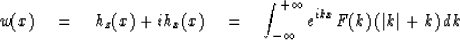and that F(k) (|k| + k) is a one-sided function of k. With a total field magnetometer one observes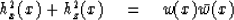What can you say about getting F(k) from this?

(d)
How unique are hx(x) and hz(x) if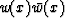is given?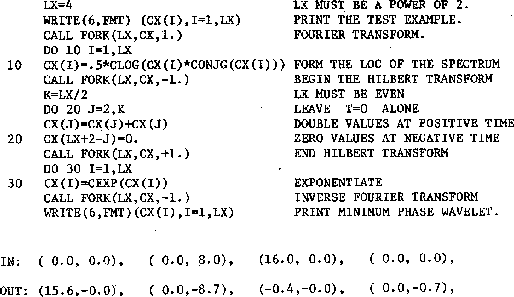3-6
Figure 6
A program to do spectral factorization by means of fast Fourier transform and Hilbert transform. Complex arithmetic is mandatory. Results are approximate since integration around the unit circle has been approximated by summation over four points.Next: CAUSALITY AND WAVE PROPAGATION Up: Spectral factorization Previous: WHITTLE'S EXP-LOG METHOD
Stanford Exploration Project
10/30/1997